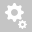##Recently viewed tickets# ko and self weight anayses in sloped ground

Question:

Dear Midas Expert

GTSNX user Nis company has a query about defining initial stress in sloped ground.

I found some explanation from manual in user manunal chapter analysis- page 473. However I couldnt understand how to activate initial stage for sloped ground in the correct way.

I attached the manual  engineering example file  given as with and without initial stress option.
Can you please send the gts nx file of these two simulations  so that we can understand how to model initial stage of these 2 cases.

Dear Customer,

Do you mean initial stage for stress analysis from analysis control?

If yes,

It will be zero for displacement at the initial stage when you tick the initial stage for stress analysis
and, you can choose "Apply K0 Condition" / "Cut-off negative effective pressure" / "Gravity Condition (If you don't choose anything)"

But, if you don't tick this initial stage for stress analysis, the displacement will be occurred at initial stage(first stage).
In this case, if you want to make the displacement to Zero condition, you need to tick the "clear displacement" at the define construction stage.

Thanks N Regards

Choi, Youngho
MIDAS Global technical center

Dear Choi,

Actually, we are asking the bahavior of GTS NX for the initial stage calculations of a sloped ground problem. Not the clear displacement item.
So please check the paragraph of my attached document in the previous message and the model samples under this paragraph.

If the Ko condition is not considered, the stress state obtained from the self-weight analysis is set as the insitu
stress. If the ground surface is horizontal, this method is the same as the K0 method when
K0=v/(1-v).  If not, a horizontal strain exists and different results than the K0 method results can be
obtained. Shear stress also occurs.
This method is generally recommended when the ground is sloped. However, because a value larger than
1 cannot be set for the K0 value, a null stage can be added for re-analysis after using the K0 method to
calculate the equilibrium stage, without adding extra external conditions when a K0 value larger than 1 is
needed. However in this case, the final equilibrium state does not satisfy the K0 condition. Also, the
modified stress is vastly different from the equilibrium point, it can be hard to calculated a converging
solution using nonlinearity.
Estimate Initial Stress of Activated Elements
In order to calculate the initial stress of ground, GTS NX perform Linear Analysis even if nonlinear material
is assigned to the elements. In this case, it can result in, sometimes, over-estimating the soil behavior
(large displacement). Initial Stress Options can eliminate this problem especially for newly activated
elements which are to simulate a fill-up ground such as backfill and embankment.

So we need a detailed answer for explaining this phenomena in GTS NX.